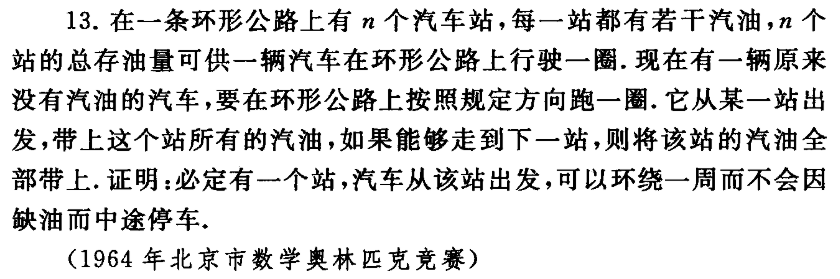# PKUSC2018游记

——题记

## 6月2日 Day 1 数学考试+机试1

1. 因式分解$bc(b+c)+ac(c-a)-ab(a+b)$
2. $n$个数，两两乘积之和为$1$。删去其中一个数，证明一定存在一种删数方案使得剩下数之和不超过$\sqrt2$
3. $i=\sqrt{-1}$，求满足$(1+i)^n=(1-i)^n$的最小正整数$n$
4. 一个环形道路上有$n$个加油站，每个加油站的油量之和足够你开车沿道路行驶一周。每当你经过一个加油站，你就能获得这个加油站中的所有油。初始时车上没有任何油。求证：一定存在至少一个加油站，使得你从那里出发能中途不缺油地完整沿道路行驶一周。
5. $2\sqrt{2y-1}=y^3+1$的所有实数解。
6. 已知$m$是大于$2$的正奇数，求最小的正整数$n$满足$m^n-1$能被$2^{2018}$整除。
7. 计算$4\arctan\frac15-\arctan\frac1{239}$
8. 在三角形内选择一点，过该点引$3$条与三角形三边平行的直线，形成的$3$个小三角形的面积分别为$S_1,S_2,S_3$。求原三角形的面积。
9. 一个正$n$棱锥，底面边长为$q$，侧面棱长为$a$，求其内切球半径$R$
10. 有一个长度为$n(n=2k+1)$的序列，序列中只包含01，问最少比较几次能找到序列上的一个位置，满足这个位置的数在序列中的出现次数至少是$k+1$

T2感觉40分和100分做法复杂度应该都是$2^n$乘上个什么东西，但又想不出枚举的是什么意义。

T3题目看反，百思不得其解，在心里骂了一万遍出题人。

## 6月3日 Day 2 面试+机试2

• 面试官1：

• 你作为初三选手，为什么想到要来北京大学？
• 介绍你的三个优点和三个缺点？
• 你父母是做什么工作的？
• 你父母做的都是与计算机无关的专业，为什么你会选择学习计算机？
• 你学习上是否遇到过什么困难？如何解决的？
• 面试官2：

• 能否向我介绍一下你的中学？初中和高中？
• 为什么想到要来PKUSC，你认为你能从中有什么收获？
• 你对你初三选手的身份有什么感想？
• 你父母在你的成长中对你又有什么帮助？
• 你学了这么长时间编程，是否有想过开发一些实用的项目？
• 你是否有和其他人合作开发过一些项目？
• 面试官3：

• Could you introduce yourself to me?
• Do you know something about AI?
我：可以说中文吗？
• 那你是否知道它们是如何被实现的呢？

T1的20分暴力和T2的8分暴力一看就很好写，果断先打完。T3一直搞不懂怎样实现多边形的旋转，30分的也不会写。

T1用自己的假结论和暴力拍了一下找反例，发现好像如果行动的方向会变，那么一定只会换一次方向。尝试着自己证了一下，也比较简单。写了一发$O(n^2\log n+q)$的线段树做法45分。把线段树换成后缀$\min$就70分了。看了一下100分的范围是$n,q\le3\times10^5$，感觉有$\mathcal O(n\log n)$$\mathcal O(n\log^2 n)$之类的做法，但是想了半天还是不会做。

## 6月28日 Day 27 停课学数竞

（《命题人讲座 图论》P83）（然而这跟图论又有什么关系？）

posted @ 2018-06-02 22:06  skylee03  阅读(1683)  评论(16编辑  收藏